Showing first {{hits.length}} results of {{hits_total}} for {{searchQueryText}}{{hits.length}} results for {{searchQueryText}}

There are a few LaTeX packages to create chemistry formulae: chemfig, ochem, streetex, and xymtex. The most intuitive is probably the chemfig package. This article explains how to use the chemfig package to create chemical formulas in LaTeX. Contents 1 Introduction 2 Angles 3 Rings 4 Branches 5 Customizing the formulas 6 Reference guide 7 Further reading Introduction Drawing a molecule consists mainly of connecting groups of atoms with lines. Simple linear formulas can be easily drawn with chemfig, let's see: \documentclass{article} \usepackage[utf8]{inputenc} \usepackage[english]{babel} \usepackage{chemfig} \section{Introduction} Writing chemical formulae with chemfig is straightforward. \chemfig{O=H} \end{document}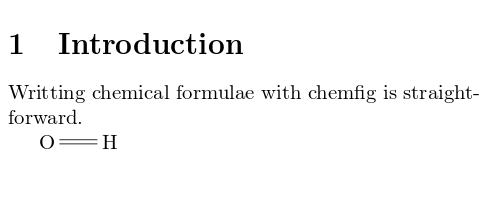The package is imported by \usepackage{chemfig} in the preamble. The command \chemfig{O=H} the draws the molecule. The symbol = determines the type of bond. See the reference guide for a list of bond types.  Open an example of the chemfig package in Overleaf Angles There are several ways to define angles to draw the bonds between molecules. To define chemical formulae you can use units that define the angles \chemfig{A-B-C} Absolute angles \chemfig{A-[:50]B-[:-25]C} Relative angles \chemfig{A-[::50]B-[::-25]C}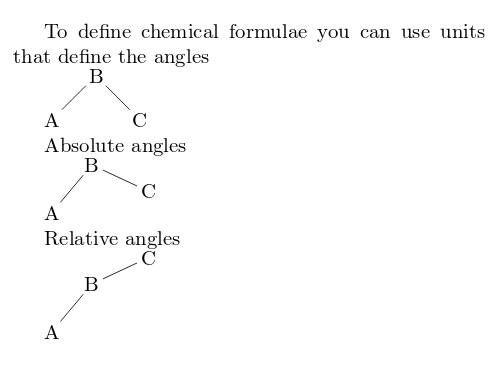Each one of the three commands in the example above uses a different method to determine the angle between bonds. default units In the command \chemfig{A-B-C} the parameters inside brackets set the angle in special units, each unit equals 45°. Hence in the example the angles are 45° and 315°. absolute units The angles can be set in absolute units, in the command \chemfig{A-[:50]B-[:-25]C} the parameter inside the brackets represent the angle, in degrees, measured from the horizontal baseline. Negative angles are allowed. relative angles In the third example \chemfig{A-[::50]B-[::-25]C} the angles are measured from the previous bond, instead of the baseline.  Open an example of the chemfig package in Overleaf Rings The example below presents the syntax to draw regular polygons Regular polygons \chemfig{A*5(-B=C-D-E=)} Incomplete rings are also possible \chemfig{A*5(-B=C-D)}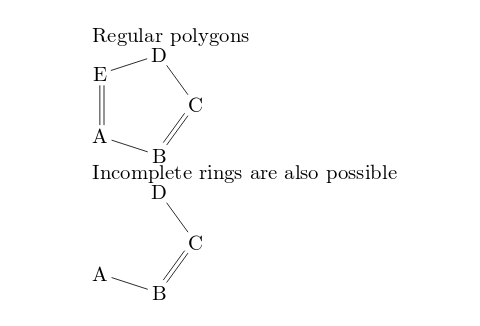The syntax of the command \chemfig{A*5(-B=C-D-E=)} is explained below: A This is the first atom, the rest of the atoms will be drawn from here *5 Number of sides of the polygon (-B=C-D-E=) The rest of the atoms and bonds. If not enough are passed to complete the polygon, an incomplete ring will be drawn. There is an additional parameter that can be passed to this command, a double asterisk. For instance, if "**5" is set instead of "*5" a circle is drawn inside the polygon.  Open an example of the chemfig package in Overleaf Branches Chemical formulas are not always linear, branched formulas are actually the most common type. Below an example on how to create them. Branched molecule \vspace{.5cm} \chemfig{H-C(-H)(-H)-C(=O)-H}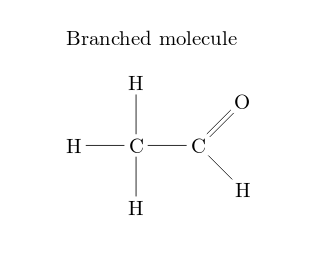Branches in each node are created by adding formulas inside parentheses. For instance, the code C(-H)(-H) creates two branches in "C", one with a 2 units angle (90°) and other with a 6 units angle (270°). Branches can also be added to rings Branched ring \vspace{.5cm} \chemfig{A*6(-B=C(-CH_3)-D-E-F(=G)=)}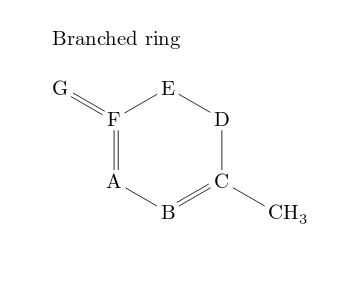The syntax is similar, using parentheses a branch can be attached to a node (atom). For instance F(=G) attaches a branch to the node "F". More complex examples can be created using nested branches and even attaching rings as branches.  Open an example of the chemfig package in Overleaf Customizing the formulas Several parameters such as colours and the node separation can be changed, also additional text to describe the formula can be added. {\huge \setbondstyle{red,line width=2pt,dash pattern=on 2pt off 2pt} \setatomsep{2em} \chemname {\chemfig{H-C(-H)(-H)-C(=O)-H}} {Ethanal} }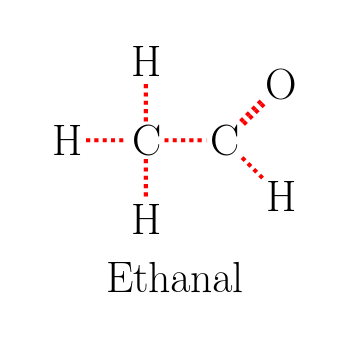There are three new command here: \setbondstyle{ } Inside the braces several style-related commands can be passed using the tikz syntax. \setatomstep{2em} The separation between atoms (nodes) in the formula is set to 2em. Other LaTeX units can be used. \chemname{}{} The first parameter in this command is a chemfig formula, the second one is some text that will be printed below the formula. In the example the text is "Ethanal". Notice also that the font used is huge. You can use any other font size and the formula will be scaled accordingly.  Open an example of the chemfig package in Overleaf Reference guide Bond types Bond type code output single \chemfig{O - H}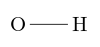double \chemfig{O = H}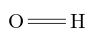triple \chemfig{O ~ H}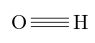plain right cram \chemfig{O > H}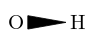plain left cram \chemfig{O < H}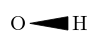dashed right cram \chemfig{O >: H}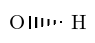dashed left cram \chemfig{O <: H}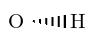hollow right cram \chemfig{O >| H}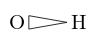hollow left cram \chemfig{O <| H}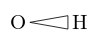Further reading For more information see Molecular orbital diagrams Feynman diagrams TikZ package Drawing Diagrams Directly in LaTeX Inserting Images List of Greek letters and math symbols The chemfig package documentation The tikz/pgf package documentation